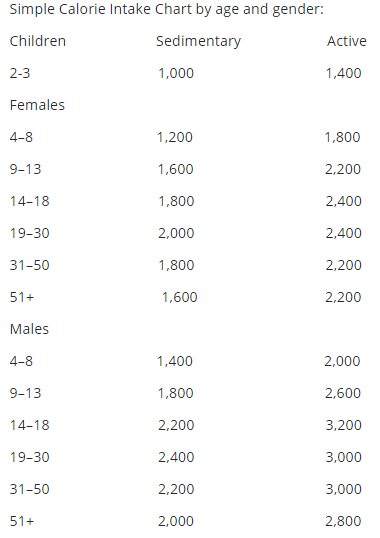If you want to figure out exactly what your calories are for each day follow the steps that are listed below.

Step 1:

Calculate your BMR using the Harris-Benedict principle formula.

Women: BMR = 655 + ( 4.30 x weight in pounds ) + ( 4.7 x height in inches ) – ( 4.7 x age in years )

Men: BMR = 66 + ( 6.3 x weight in pounds ) + ( 12.7 x height in inches ) – ( 6.8 x age in year )

This is the calories your body needs to sustain normal function. In this example the BMR (Basal Metabolic Rate) is around 1,635 calories each day.

Example: Men

1. 6.3 x 136 lbs = 856.8

2. 12.7 x 70 height in inches = 889

3. 6.8 x 26 years of age = 176.8

4. 66 + 856.8 + 889 – 176.8 = 1,635 (Your BMR)

Step 2:

Now what you want to do is to determine your activity level and then multiply it with your BMR.

Sedimentary: 20%

Lightly Active: 30%

Moderately Active (You exercise 4 or more days of the week): 40%

Very Active (You exercise 6 or 7 day in a week): 50%

Extra Active (You do hard labor or are in athletic training): 60%

Example:

1,635 calories x .60 (60%) = 981 calories + 1,635 (Your BMR) = 2,616

Now your total calories needed each day are 2,616